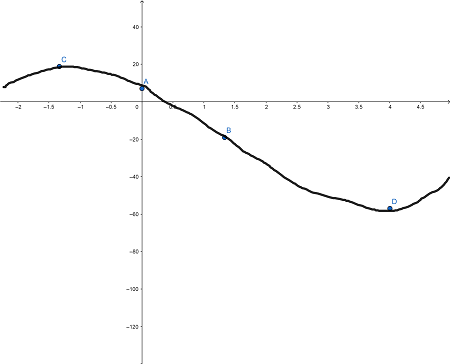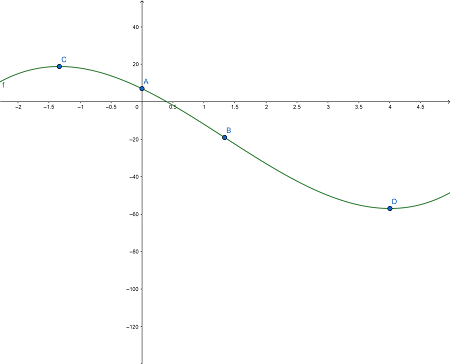# Sketch the graph of the given function by determining the appropriate information and points from...

## Question:

Sketch the graph of the given function by determining the appropriate information and points from the first and second derivatives. Use a graphing calculator to check the graph.

{eq}y = x^3-4x^2- 16x + 7 {/eq}

## Increasing/Decreasing and Concavity:

The first and second derivative can tell us a lot about the shape of a function. We recall the following

1) If {eq}f'(x)>0 {/eq} on an interval {eq}I {/eq} then {eq}f(x) {/eq} is increasing on {eq}I {/eq}.

2) If {eq}f'(x)<0 {/eq} on an interval {eq}I {/eq} then {eq}f(x) {/eq} is decreasing on {eq}I. {/eq}

3) If {eq}f''(x)>0 {/eq} on an interval {eq}I {/eq}, then {eq}f(x) {/eq} is concave up on {eq}I. {/eq}

4) If {eq}f''(x)<0 {/eq} on an interval {eq}I, {/eq} then {eq}f(x) {/eq} is concave down on {eq}I. {/eq}

We also recall the following:

1) If {eq}f(x) {/eq} is continuous at {eq}x=a {/eq} and f changes from increasing to decreasing (decreasing to increasing) at {eq}x=a, {/eq} then {eq}f {/eq} has a local maximum (minimum) at {eq}x=a. {/eq}

If {eq}f(x) {/eq} is continuous at {eq}x=a {/eq} and {eq}f(x) {/eq} changes concavity at {eq}x=a, {/eq} then {eq}f(x) {/eq} has an inflection point at {eq}x=a. {/eq}

Let {eq}f(x)=x^3-4x^2-16x+7 {/eq}. We first note that {eq}f(0)=7 {/eq} meaning that {eq}f(x) {/eq} has a {eq}y {/eq}-intercept at {eq}y=7 {/eq}. The {eq}x {/eq}-intercepts are not obvious, so we don't compute. Next we note that {eq}\displaystyle \lim_{x\to \ \infty} f(x)=\infty {/eq} and {eq}\displaystyle \lim_{x\to \ -\infty} f(x)=-\infty {/eq}. Computing the derivatives we have

{eq}f'(x)=3x^2-8x-16=(3x+4)(x-4)\\ f''(x)=6x-8=2(3x-4) {/eq}

Setting {eq}f'(x)=0 {/eq} we have

{eq}x=\frac{-4}{3} {/eq} and {eq}x=4 {/eq}.

We then note that on {eq}(-\infty,-4/3),\, f'(x)>0 {/eq}, on {eq}(-4/3,4),\, f'(x)<0 {/eq} and on {eq}(4,\infty),\, f'(x)>0 {/eq}. So {eq}f(x) {/eq} is increasing on {eq}(-\infty,-4/3) {/eq} and on {eq}(4,\infty) {/eq} and is decreasing on {eq}(-4/3,4) {/eq}.

This gives a local maximum at {eq}(-4/3,f(-4/3))=(-4/3,509/27) {/eq} and a local maximum at {eq}(4,f(4))=(4,-57) {/eq}

Next we note that {eq}f''(x)=0 {/eq} for {eq}x=\frac{4}{3} {/eq}. On {eq}(-\infty,4/3),\, f''(x)<0 {/eq} and on {eq}(4/3,\infty),\, f''(x)>0 {/eq}. So {eq}f(x) {/eq} is concave down on {eq}(-\infty,4/3) {/eq} and concave up on {eq}(4/3,\infty) {/eq}. We also have {eq}f(4/3)=\frac{-515}{27} {/eq}

The local maximum, minimum, {eq}y {/eq}-intercept and inflection point are shown on the graph below and then a hand sketch of the curve is shown followed by a computer generated sketch.